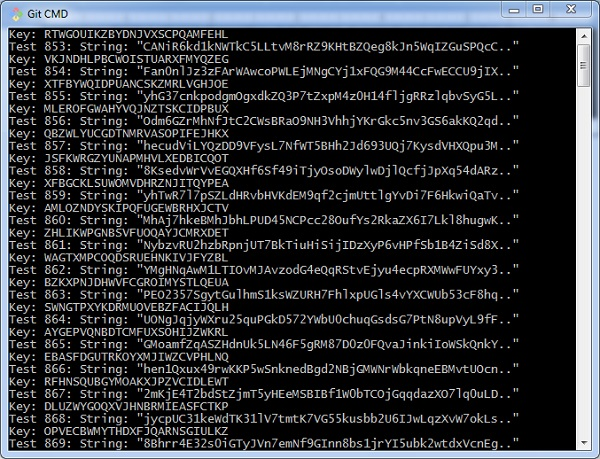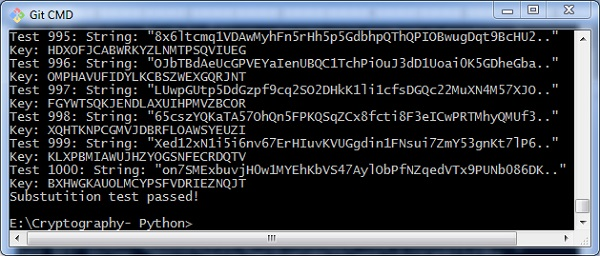# Testing of Simple Substitution Cipher

In this chapter, we will focus on testing substitution cipher using various methods, which helps to generate random strings as given below −

import random, string, substitution
def main():
for i in range(1000):
key = substitution.getRandomKey()
message = random_string()
print('Test %s: String: "%s.."' % (i + 1, message[:50]))
print("Key: " + key)
encrypted = substitution.translateMessage(message, key, 'E')
decrypted = substitution.translateMessage(encrypted, key, 'D')

if decrypted != message:
print('ERROR: Decrypted: "%s" Key: %s' % (decrypted, key))
sys.exit()
print('Substutition test passed!')

def random_string(size = 5000, chars = string.ascii_letters + string.digits):
return ''.join(random.choice(chars) for _ in range(size))
if __name__ == '__main__':
main()


## Output

You can observe the output as randomly generated strings which helps in generating random plain text messages, as shown below −After the test is successfully completed, we can observe the output message Substitution test passed!.Thus, you can hack a substitution cipher in the systematic manner.